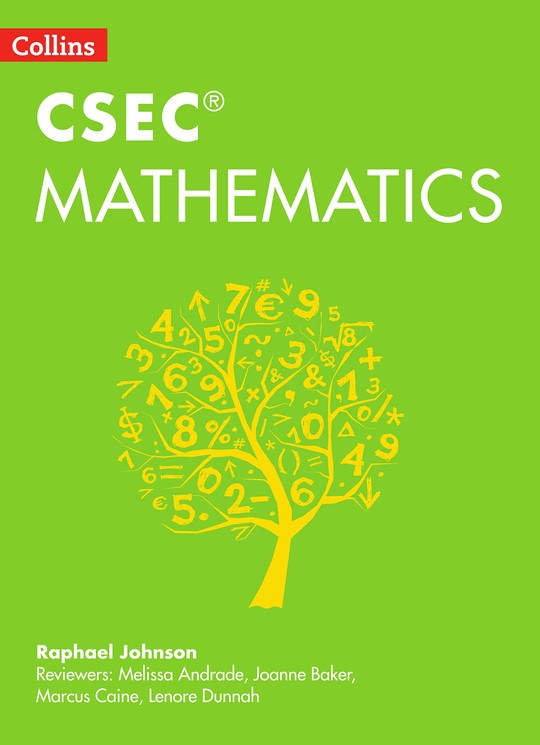# Collins CSEC® Mathematics

By Raphael Johnson
Book Description

Collins CSEC(R) Mathematics provides a new approach to the study of the CSEC(R) mathematics syllabus. Fully grounded in the practical application of maths in the world today, it features clear explanations, differentiated practice exercises and guidance on the School Based Assessment.Collins CSEC(R) Mathematics has been written for the CXC(R) syllabus effective for examinations from May-June 2018. It provides full coverage of this syllabus, with real-world and everyday contexts for all topics to help Caribbean students understand why maths is so important for the world today, with guidance on the School Based Assessment, worked examples, and differentiated - or graded - practice questions to help students understand the level they are working at and the level they need to be working at to achieve a good grade. Written by a highly experienced teacher in the Caribbean.

• Cover
• Title
• Collins
• Contents
• Introduction
• How to use this book
• 1 Number theory and Computation
• 1.1 History of numbers
• 1.2 Classification of numbers
• 1.3 Ordering a set of real numbers
• 1.4 Place value of whole numbers
• 1.5 Revision: Addition, subtraction, multiplication and division
• 1.6 Defined arithmetic operations
• 1.7 Associativity, commutativity and distributivity
• 1.8 Identity for addition and multiplication
• 1.9 Additive and multiplicative inverses
• 1.10 Multiplication and division involving zero
• 1.11 Order of arithmetic operations (BODMAS)
• 1.12 Powers of numbers
• 1.13 Reciprocal of a number
• 1.14 Factors and multiples
• 1.15 Fractions: revision
• 1.16 Operations with fractions
• 1.17 Decimal fractions: revision
• 1.18 Operations with decimals
• 1.19 Approximating numbers
• 1.20 Standard form (scientific notation
• 1.21 Mixed operations with decimals
• 1.22 Percentages
• 1.23 Conversion between units of measure
• 1.24 Ratio
• 1.25 Direct and indirect proportion
• 1.26 Rates
• 1.27 Arithmetic mean (average
• 1.28 Sequences
• 1.29 Number bases
• 1.30 Operations with binary numbers
• 1.31 Base 4 and base 8
• 1.32 The use of calculators innumber theory and computation
• End of chapter summary
• Examination-type questions for Chapter 1
• 2 Consumer arithmetic
• 2.1 Discount, sales tax, profit and loss
• 2.2 Simple and compound interest
• 2.3 Hire purchase and mortgages
• 2.4 Rates and taxes
• 2.5 Utilities payments
• 2.6 Currency and foreign exchange
• 2.7 Salaries and wages
• 2.8 Income tax
• 2.9 Insurance
• End of chapter summary
• Examination-type questions for Chapter 2
• 3 Algebra
• 3.1 Directed numbers and arithmetic operations on them
• 3.2 The meaning and importance of algebra
• 3.3 Algebraic notation
• 3.4 Laws of indices
• 3.5 Using the four basic operations to simplify algebraic expressions
• 3.6 Algebraic fractions
• 3.7 Substitution
• 3.8 Binary operations
• 3.9 Using the distributive law to expand algebraic expressions
• 3.10 Simple factorisation of an algebraic expression
• 3.11 Simple linear equations in one unknown
• 3.12 Inequalities
• 3.13 Transposition - changing the subject of a formula
• 3.14 Simultaneous linear equations in two unknowns
• 3.15 Factorising by grouping
• 3.18 Simultaneous equations: one quadratic and one linear
• 3.19 Identities and equations - what is the difference?
• 3.20 Direct and inverse variation
• End of chapter summary
• Examination-type questions for Chapter 3
• 4 Geometry and trigonometry
• 4.1 Geometrical concepts
• 4.2 Drawing and measuring line sefments and angles
• 4.3 Constructing lines, angles and polygons
• 4.4 Symmetry in plane figures
• 4.5 Properties of angles, lines and polygons
• 4.6 Three-dimensional shapes
• 4.7 Circles
• 4.8 Transformation geometry
• 4.9 Trigonometry
• 4.10 Spatial geometry: projections and scale drawing
• 4.11 Trigonometry in three dimensions
• End of chapter summary
• Examination-type questions for Chapter 4
• 5 Measurement
• 5.1 Quantities and units
• 5.2 Length
• 5.3 Mass
• 5.4 More units of length and mass
• 5.5 Time
• 5.6 Weight
• 5.7 Speed
• 5.8 Density
• 5.9 Perimeter
• 5.10 Area
• 5.11 Margin of error in measurement
• 5.12 Scale drawing
• 5.13 Volume
• 5.14 Surface areas of solids
• End of chapter summary
• Examination-type questions for Chapter 5
• 6 Sets
• 6.1 Definition of a set and related concepts
• 6.2 Intersection, union and complements of not more than 3 sets
• 6.3 Venn diagrams
• 6.4 Using set theory to solve problems in number theory, algebra and geometry
• End of chapter summary
• Examination-type questions for Chapter 6
• 7 Relations, functions and graphs
• 7.1 Relations and functions
• 7.2 Coordinate geometry and graphs of linear functions
• 7.3 Linear inequalities
• 7.5 Graphs of other functions
• End of chapter summary
• Examination-type questions for Chapter 7
• 8 Statistics
• 8.1 Sample statistics and population parameters
• 8.2 Classifying and representing data
• 8.3 Statistical diagrams
• 8.4 Measures of central tendency
• 8.5 Probability
• End of chapter summary
• Examination-type questions for Chapter 8
• 9 Vectors, matrices and transformations
• 9.1 Concepts associated with vectors
• 9.2 Position vectors
• 9.3 Matrices
• 9.4 Vectors, transformations and matrices
• End of chapter summary
• Examination-type questions for Chapter 9
• 10 T he School-Based Assessment in Mathematics
• Introduction
• Managing the project
• Devising a project topic
• Collecting data
• Problem-solving strategies
• Project report
• Project mark scheme
• Sample Mathematics SBA
• Examples of SBA topics
• 11 Examination Practice
• Examination 1
• Examination 2
• Examination 3
• Using the calculator in the mathematics classroom
• 13 Teaching notes
• Exercises
• Problems: examples, strategies and solutions
• Math investigations - examples
• The use of calculators
• In support of the use of calculators
• Criticisms of the use of the calculator
• Manipulatives and teaching aids